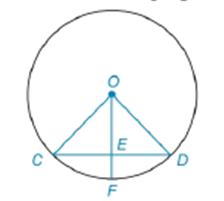Chapter 6.3, Problem 2EElementary Geometry For College St...

7th Edition
Alexander + 2 others
ISBN: 9781337614085

Solutions

Chapter
SectionElementary Geometry For College St...

7th Edition
Alexander + 2 others
ISBN: 9781337614085
Textbook Problem

Note: Exercises preceded by an asterisk are of a more challenging nature.Given: O C = 8     a n d     O E = 6 O E ¯ ⊥ C D ¯     i n     ⊙ O Find: C DTo determine

To find:

To find CD.

Explanation

Given that OC=8 and OE=6 and OE¯CD¯ in O

Consider the following figure,

Given that OE¯CD¯ in O then ΔOCE is right triangle.

Pythagorean theorem:

In a right angled triangle then the square of the hypotenuse is equal to the sum of the squares of the other two sides.

Consider the ΔOCE to get the following,

OC2=OE2+CE2

Substitute the value of OC=8 and OE=6 to get the following,

Still sussing out bartleby?

Check out a sample textbook solution.

See a sample solution

The Solution to Your Study Problems

Bartleby provides explanations to thousands of textbook problems written by our experts, many with advanced degrees!

Get Started

Write each of the following as radicals.

Mathematical Applications for the Management, Life, and Social Sciences

Find all possible real solutions of each equation in Exercises 3144. y32y22y3=0

Finite Mathematics and Applied Calculus (MindTap Course List)

Evaluate the integral. 33. 32xx2dx

Single Variable Calculus: Early Transcendentals

The implied domain of is: (1, ∞) (−∞, 1) x ≠ 1 (−1, 1)

Study Guide for Stewart's Single Variable Calculus: Early Transcendentals, 8th

The quadratic approximation for at a = 1 is:

Study Guide for Stewart's Multivariable Calculus, 8th